# Introducing Generative Recursion CS 5010 Program Design Paradigms

• Slides: 23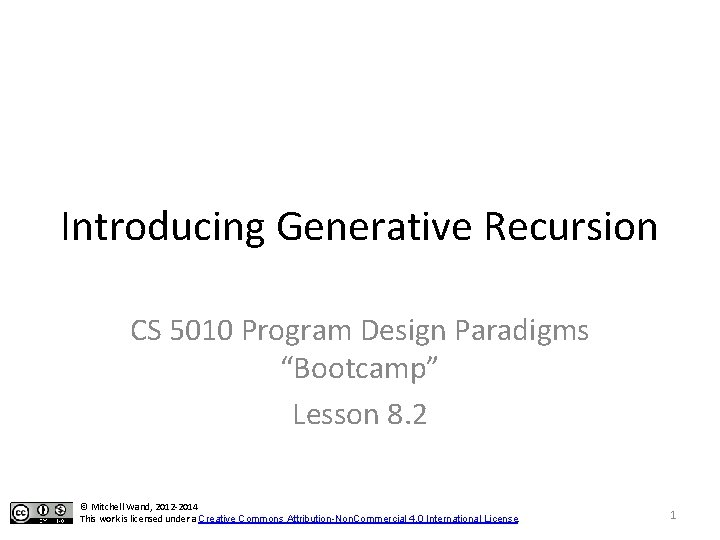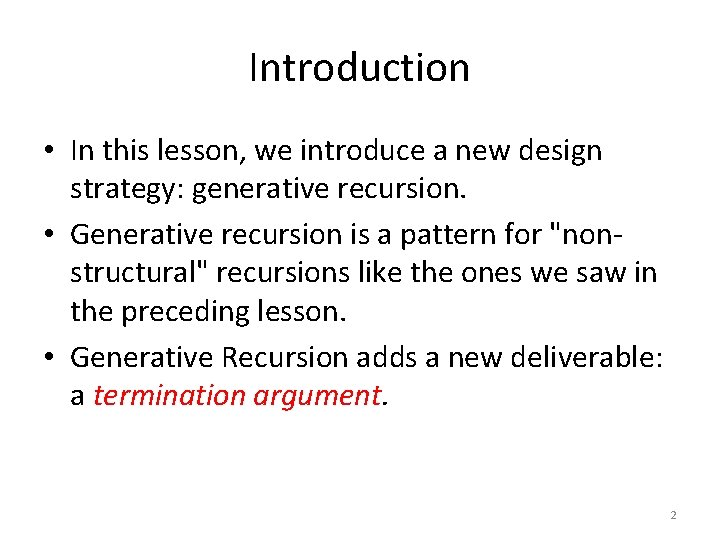Introduction • In this lesson, we introduce a new design strategy: generative recursion. • Generative recursion is a pattern for "nonstructural" recursions like the ones we saw in the preceding lesson. • Generative Recursion adds a new deliverable: a termination argument. 2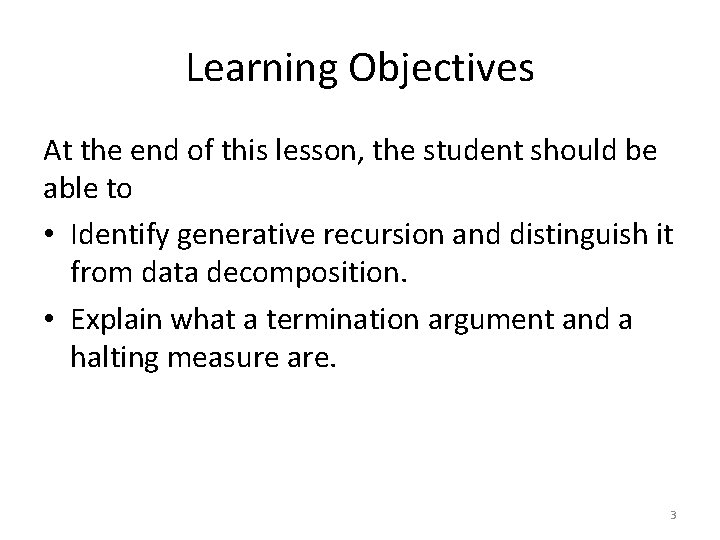Learning Objectives At the end of this lesson, the student should be able to • Identify generative recursion and distinguish it from data decomposition. • Explain what a termination argument and a halting measure are. 3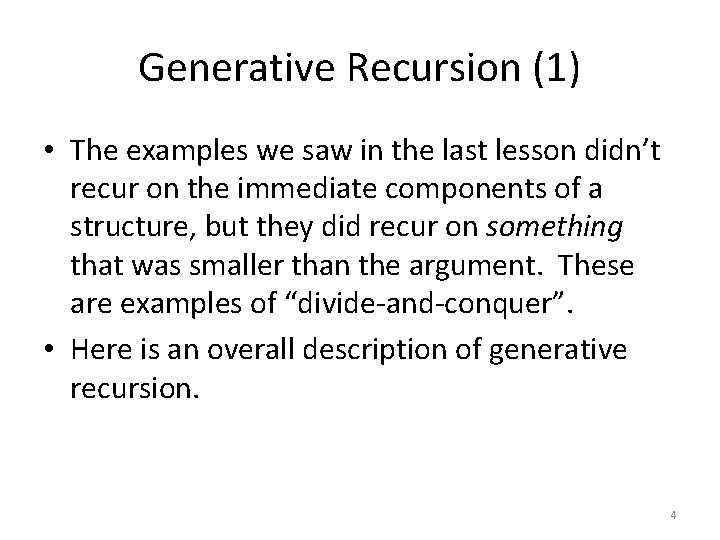Generative Recursion (1) • The examples we saw in the last lesson didn’t recur on the immediate components of a structure, but they did recur on something that was smaller than the argument. These are examples of “divide-and-conquer”. • Here is an overall description of generative recursion. 4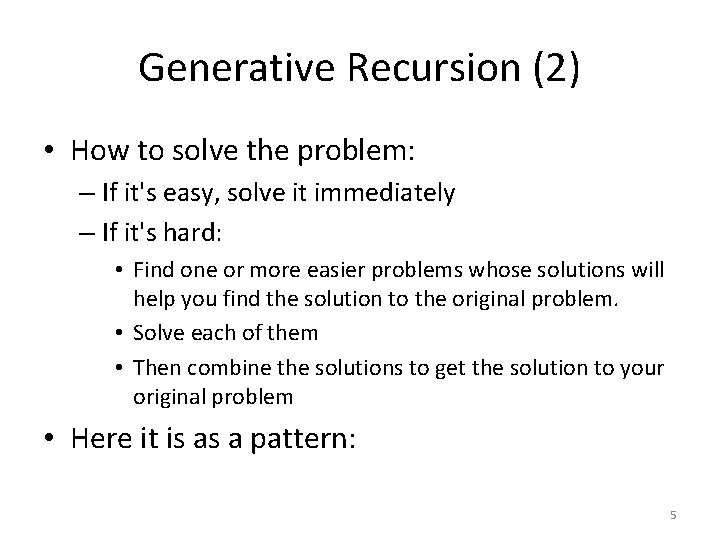Generative Recursion (2) • How to solve the problem: – If it's easy, solve it immediately – If it's hard: • Find one or more easier problems whose solutions will help you find the solution to the original problem. • Solve each of them • Then combine the solutions to get the solution to your original problem • Here it is as a pattern: 5Pattern for Generative Recursion (1) ; ; solve : Problem -> Solution ; ; purpose statement. . . ; ; TERMINATION ARGUMENT: explain why new-problem 1 and newproblem 2 are easier than the-problem. (define (solve the-problem) (cond [(trivial 1? the-problem) (trivial-solution 1 the-problem)] [(trivial 2? the-problem) (trivial-solution 2 the-problem)] [(difficult? the-problem) (local ((define new-problem 1 (divider 1 the-problem)) (define new-problem 2 (divider 2 the-problem)))) (combine-solutions (solve new-problem 1) There is no magic recipe for finding easier (solve new-problem 2))])) subproblems. You must understand the structure of the problem domain. 6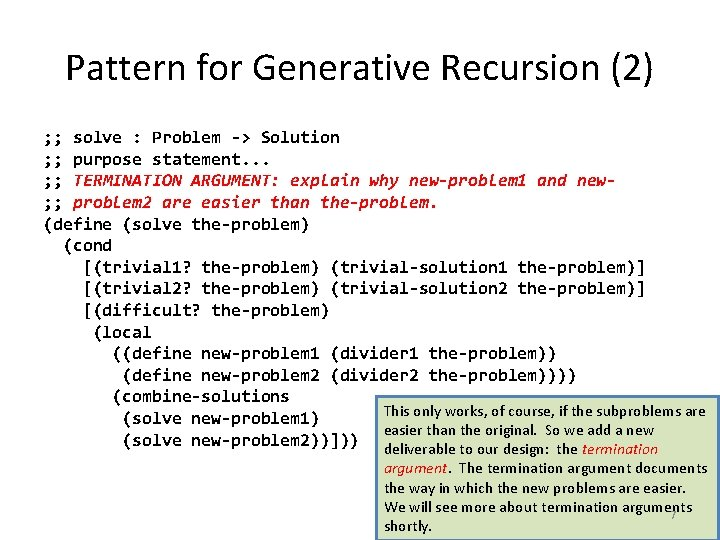Pattern for Generative Recursion (2) ; ; solve : Problem -> Solution ; ; purpose statement. . . ; ; TERMINATION ARGUMENT: explain why new-problem 1 and new; ; problem 2 are easier than the-problem. (define (solve the-problem) (cond [(trivial 1? the-problem) (trivial-solution 1 the-problem)] [(trivial 2? the-problem) (trivial-solution 2 the-problem)] [(difficult? the-problem) (local ((define new-problem 1 (divider 1 the-problem)) (define new-problem 2 (divider 2 the-problem)))) (combine-solutions This only works, of course, if the subproblems are (solve new-problem 1) easier than the original. So we add a new (solve new-problem 2))])) deliverable to our design: the termination argument. The termination argument documents the way in which the new problems are easier. We will see more about termination arguments 7 shortly.This pattern is more flexible than a template • How many trivial cases are there? • How many subproblems do you divide your original into? – Could be just 1 – Could be a whole list We say “pattern” rather than “template” because we intend the pattern to be more flexible than our templates have been. We might have different numbers of trivial cases, or different numbers of subproblems. Let’s look at some variations 8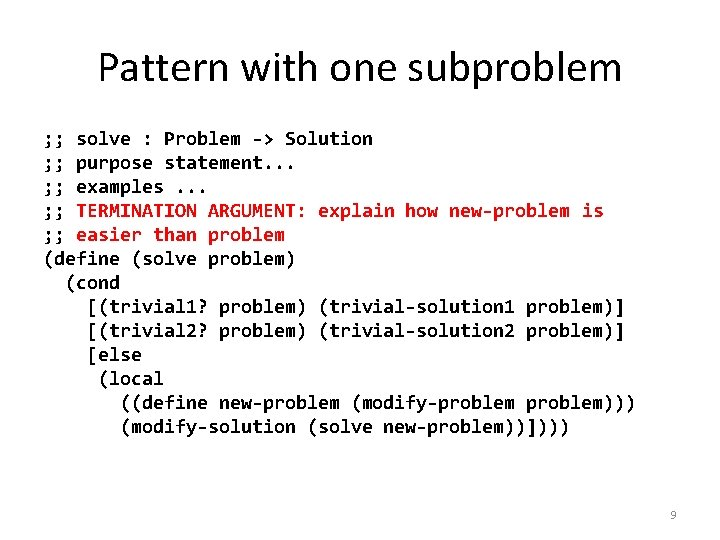Pattern with one subproblem ; ; solve : Problem -> Solution ; ; purpose statement. . . ; ; examples. . . ; ; TERMINATION ARGUMENT: explain how new-problem is ; ; easier than problem (define (solve problem) (cond [(trivial 1? problem) (trivial-solution 1 problem)] [(trivial 2? problem) (trivial-solution 2 problem)] [else (local ((define new-problem (modify-problem))) (modify-solution (solve new-problem))]))) 9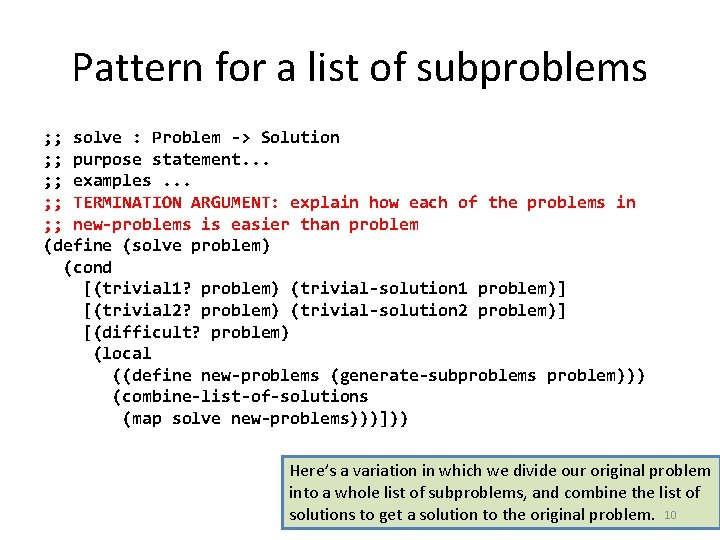Pattern for a list of subproblems ; ; solve : Problem -> Solution ; ; purpose statement. . . ; ; examples. . . ; ; TERMINATION ARGUMENT: explain how each of the problems in ; ; new-problems is easier than problem (define (solve problem) (cond [(trivial 1? problem) (trivial-solution 1 problem)] [(trivial 2? problem) (trivial-solution 2 problem)] [(difficult? problem) (local ((define new-problems (generate-subproblems problem))) (combine-list-of-solutions (map solve new-problems)))])) Here’s a variation in which we divide our original problem into a whole list of subproblems, and combine the list of solutions to get a solution to the original problem. 10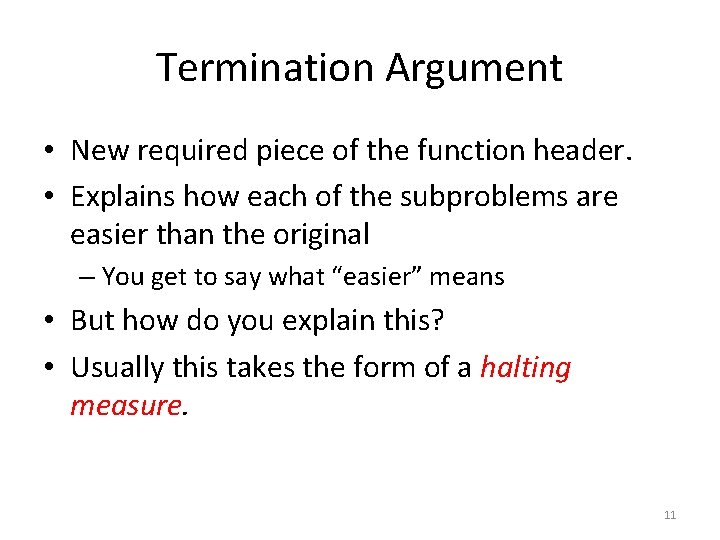Termination Argument • New required piece of the function header. • Explains how each of the subproblems are easier than the original – You get to say what “easier” means • But how do you explain this? • Usually this takes the form of a halting measure. 11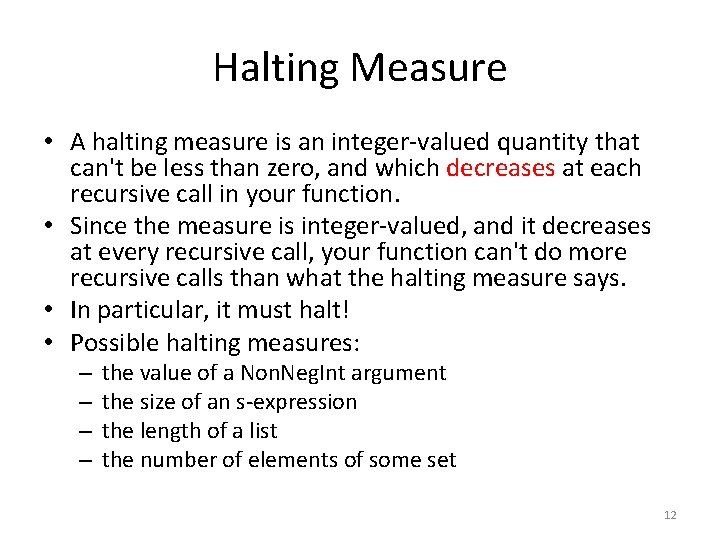Halting Measure • A halting measure is an integer-valued quantity that can't be less than zero, and which decreases at each recursive call in your function. • Since the measure is integer-valued, and it decreases at every recursive call, your function can't do more recursive calls than what the halting measure says. • In particular, it must halt! • Possible halting measures: – – the value of a Non. Neg. Int argument the size of an s-expression the length of a list the number of elements of some set 12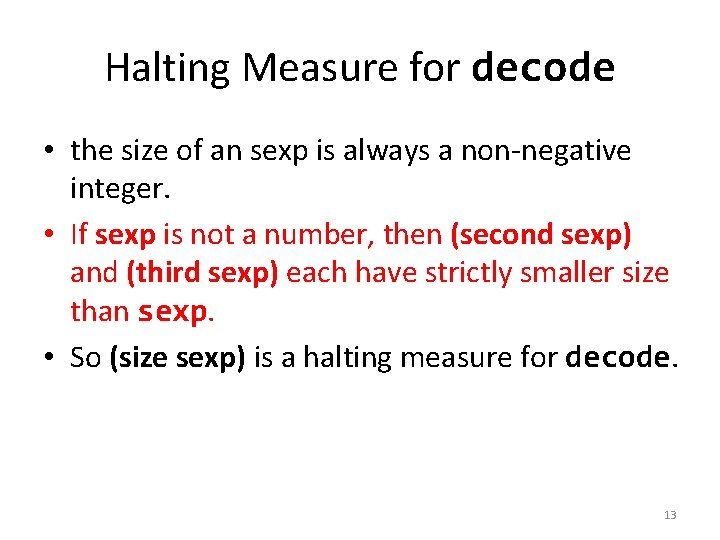Halting Measure for decode • the size of an sexp is always a non-negative integer. • If sexp is not a number, then (second sexp) and (third sexp) each have strictly smaller size than sexp. • So (size sexp) is a halting measure for decode. 13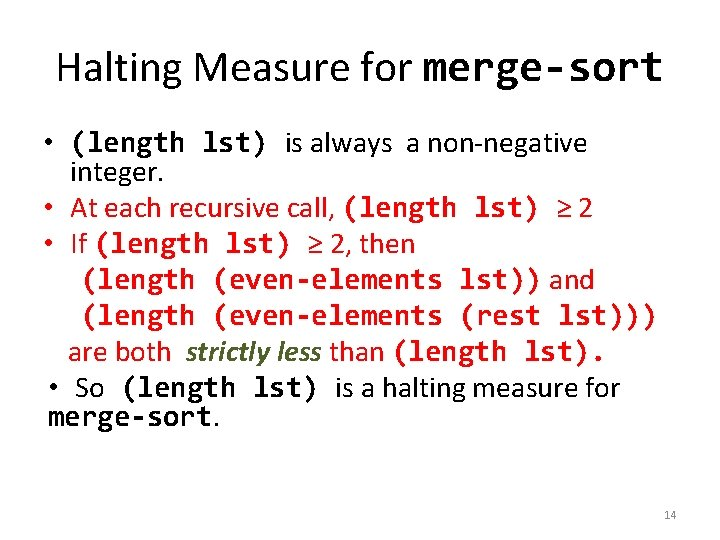Halting Measure for merge-sort • (length lst) is always a non-negative integer. • At each recursive call, (length lst) ≥ 2 • If (length lst) ≥ 2, then (length (even-elements lst)) and (length (even-elements (rest lst))) are both strictly less than (length lst). • So (length lst) is a halting measure for merge-sort. 14A Numeric Example fib : Non. Neg. Int -> Non. Neg. Int (define (fib n) (cond Here's the standard recursive definition of the fibonacci function [(= n 0) 1] [(= n 1) 1] [else (+ (fib (- n 1)) (fib (- n 2)))]))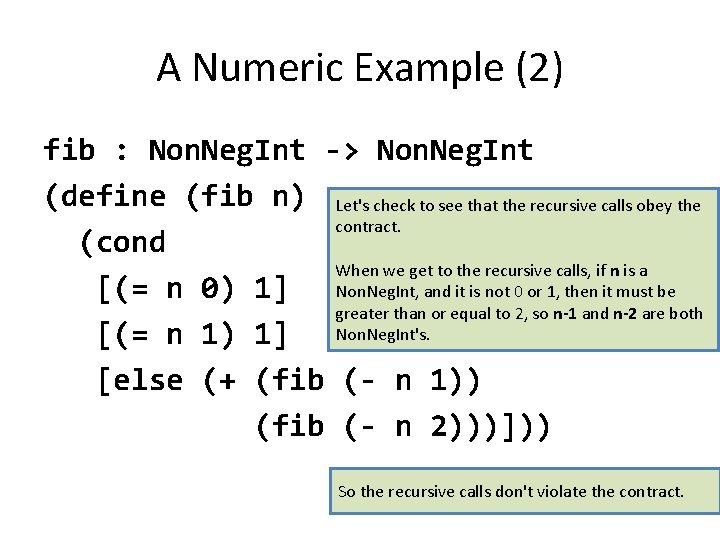A Numeric Example (2) fib : Non. Neg. Int -> Non. Neg. Int (define (fib n) Let's check to see that the recursive calls obey the contract. (cond When we get to the recursive calls, if n is a [(= n 0) 1] Non. Neg. Int, and it is not 0 or 1, then it must be greater than or equal to 2, so n-1 and n-2 are both [(= n 1) 1] Non. Neg. Int's. [else (+ (fib (- n 1)) (fib (- n 2)))])) So the recursive calls don't violate the contract.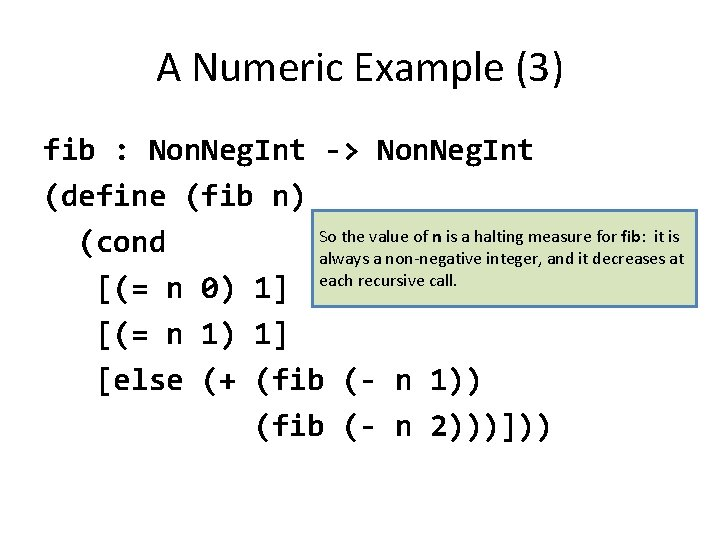A Numeric Example (3) fib : Non. Neg. Int -> Non. Neg. Int (define (fib n) So the value of n is a halting measure for fib: it is (cond always a non-negative integer, and it decreases at [(= n 0) 1] each recursive call. [(= n 1) 1] [else (+ (fib (- n 1)) (fib (- n 2)))]))What about (fib -1)? (fib -1) = (+ (fib -2) (fib -3)) = (+ (+ (fib -3) (fib -4)) (+ (fib -4) (fib -5)) = etc. Oops! This doesn't terminate!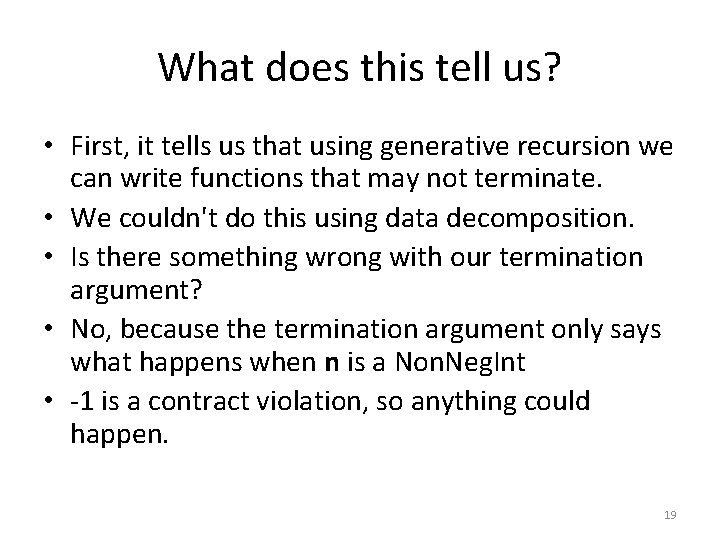What does this tell us? • First, it tells us that using generative recursion we can write functions that may not terminate. • We couldn't do this using data decomposition. • Is there something wrong with our termination argument? • No, because the termination argument only says what happens when n is a Non. Neg. Int • -1 is a contract violation, so anything could happen. 19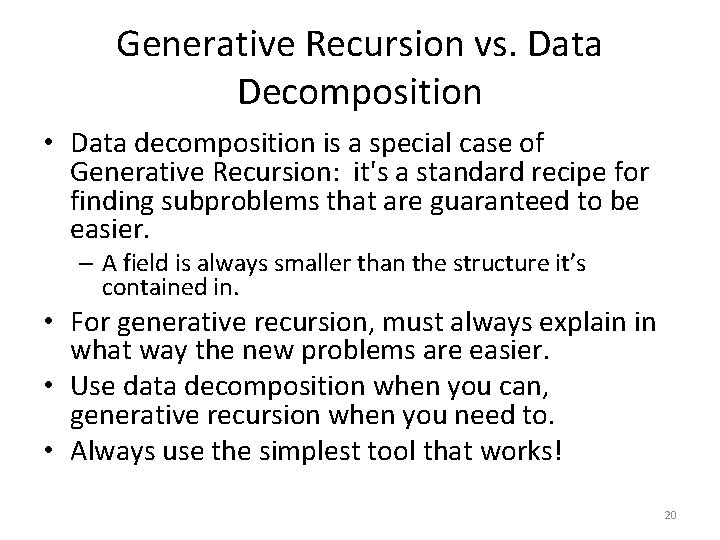Generative Recursion vs. Data Decomposition • Data decomposition is a special case of Generative Recursion: it's a standard recipe for finding subproblems that are guaranteed to be easier. – A field is always smaller than the structure it’s contained in. • For generative recursion, must always explain in what way the new problems are easier. • Use data decomposition when you can, generative recursion when you need to. • Always use the simplest tool that works! 20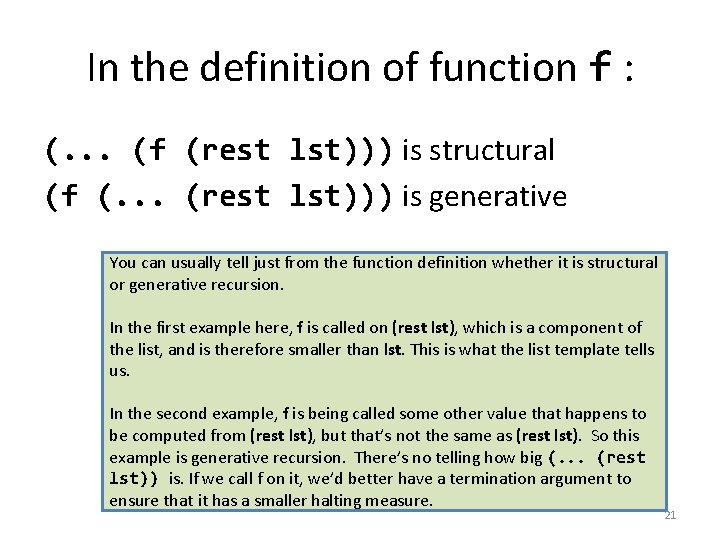In the definition of function f : (. . . (f (rest lst))) is structural (f (. . . (rest lst))) is generative You can usually tell just from the function definition whether it is structural or generative recursion. In the first example here, f is called on (rest lst), which is a component of the list, and is therefore smaller than lst. This is what the list template tells us. In the second example, f is being called some other value that happens to be computed from (rest lst), but that’s not the same as (rest lst). So this example is generative recursion. There’s no telling how big (. . . (rest lst)) is. If we call f on it, we’d better have a termination argument to ensure that it has a smaller halting measure. 21Summary (1) • We've introduced generative recursion. • Solve the problem by combining solutions to easier subproblems. • Must give a termination argument that shows why each subproblem is easier. • Easiest way to give a termination argument is by giving a halting measure. • Data decomposition is a special case where the data def guarantees the subproblem is easier. • Always use the simplest tool that works! 22Summary (2) You should now be able to • Identify generative recursion and distinguish it from data decomposition. • Explain what a termination argument and a halting measure are. 23